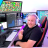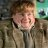# Option Greeks Calculation Labels for ThinkorSwim#### markos

##### Well-known member
VIP
Posted to TSL 7-23-19
11:45 jeffrey2360: Would be great to be able to grab live greeks in a study the same way we get price. HIstorical data is not that important, but having live ratio is very useful (delta/theta)
11:46 Mobius:
Code:
``````# Delta Calculation
# For Options with 1 dollar strikes
# Mobius
# V01.07.2019

input DayToExpiry = 2; #hint: Don't mess with this.
input Series_IV = 1;

def IV = SeriesVolatility(series = Series_IV);
def K = Floor(close);
def S = close;
def R = GetInterestRate();
def t = DayToExpiry / 365;
def d1 = (log(S / K) + ((R + (sqr(IV) / 2)) * t)) / (IV * sqrt(t));
script cnd

{

input data  = 1;

def a = AbsValue(data);

def b1 =  .31938153;

def b2 = -.356563782;

def b3 = 1.781477937;

def b4 = -1.821255978;

def b5 = 1.330274429;

def b6 =  .2316419;

def e = 1 / (1 + b6 * a);

def i = 1 - 1 / Sqrt(2 * Double.Pi) * Exp(-Power(a, 2) / 2) *

(b1 * e + b2 * e * e + b3 * Power(e, 3) + b4 * Power(e, 4) + b5 * Power(e, 5));

plot CND = if data < 0

then 1 - i

else i;

}

def Delta = cnd(d1);
AddLabel(1, "Delta Est = " + Delta, color.white);
# End Code Delta Estimate``````
11:47 Mobius: That is pretty bloody close
11:47 Mobius: usually within a penny
11:48 jeffrey2360: Oh. I got to study that code. Will it be possible to apply this to 4 options in a single study ?
11:50 Mobius: sure. You'll need to run 4 instances of the study, change the ones for puts from the above which is for calls and alter the other strikes.
11:52 Mobius: And it would help to have a degree in mathematics and coding but you can probably do it. Maybe

Last edited:
•#### mc01439

##### Well-known member
2019 Donor
VIP
Posted today on thinkScript Lounge - gift from Mobius.

Code:
``````# Greeks Calculations (originally just Delta)
# For Options
# Mobius
# V02.07.2019
# K - Option strike price
# N - Standard normal cumulative distribution function
# r - Risk free interest rate
# IV - Volatility of the underlying
# S - Price of the underlying
# t - Time to option's expiry
# Delta = N(d1)
#           d1 = (ln(S/K) + (r + (sqr(IV)/2))t) / (? (sqrt(t)))
# Gamma  = (d2) / S(IV(sqrt(t)))
# d2 =  e -(sqr(d1) / 2) / sqrt(2*pi)
# Theta = ((S d2))IV) / 2 sqrt(t)) - (rK e(rt)N(d4))
#         where phi(d3) = (exp(-(sqr(x)/2))) / (2 * sqrt(t))
#         where d4 = d1 - IV(sqrt(t))
# Vega = S phi(d1) Sqrt(t)

input DayToExpiry = 1;
input Series_IV = 1;

def IV = SeriesVolatility(series = Series_IV);
def K = if close >= Round(close / Strike_Spread, 0) * Strike_Spread
def S = close;
def r = GetInterestRate();
def t = DayToExpiry / 365;
def d1 = (Log(S / K) + ((r + (Sqr(IV) / 2)) * t)) / (IV * Sqrt(t));
addLabel(1, "Strike: " + AsDollars(K), color.white);
script N
{
input data  = 1;
def a = AbsValue(data);
def b1 =  .31938153;
def b2 = -.356563782;
def b3 = 1.781477937;
def b4 = -1.821255978;
def b5 = 1.330274429;
def b6 =  .2316419;
def e = 1 / (1 + b6 * a);
def i = 1 - 1 / Sqrt(2 * Double.Pi) * Exp(-Power(a, 2) / 2) *
(b1 * e + b2 * e * e + b3 *
Power(e, 3) + b4 * Power(e, 4) + b5 * Power(e, 5));
plot CND = if data < 0
then 1 - i
else i;
}
def Delta = N(d1);
AddLabel(1, "Delta = " + Delta, Color.WHITE);
# End Code Delta
# Gamma
def d2 = Exp(-(Sqr(d1) / 2)) / Sqrt(2 * Double.Pi);
def Gamma = d2 / (S * (IV * Sqrt(t)));
AddLabel(1, "Gamma = " + Gamma, Color.WHITE);
# End Code Gamma
# Theta
def Theta = -(-(S*d2*IV*(.5000)/
(2*sqrt(t)))-
(r*(exp(-r*t)*K))*N(d2)+(S*N(d1)*(.5000)))/365;
# (.5000) variant less than .5 e(X/t)
AddLabel(1, "Theta = " + theta, Color.WHITE);
# End Code Theta
# Vega
def Vega = (S*d2*sqrt(t))/100;
AddLabel(1, "Vega = " + Vega, color.white);
# End Code Greeks``````

••ccurtin, tradegeek, markos and 1 other person#### markos

##### Well-known member
VIP
Thanks @mc01439 ! Mobius is handing out Thanksgiving Black Friday Deals (as he called it) early. Very nice.

2

#### 2sureshk

##### Member
Posted to TSL 7-23-19
11:45 jeffrey2360: Would be great to be able to grab live greeks in a study the same way we get price. HIstorical data is not that important, but having live ratio is very useful (delta/theta)
11:46 Mobius:
Code:
``````# Delta Calculation
# For Options with 1 dollar strikes
# Mobius
# V01.07.2019

input DayToExpiry = 2; #hint: Don't mess with this.
input Series_IV = 1;

def IV = SeriesVolatility(series = Series_IV);
def K = Floor(close);
def S = close;
def R = GetInterestRate();
def t = DayToExpiry / 365;
def d1 = (log(S / K) + ((R + (sqr(IV) / 2)) * t)) / (IV * sqrt(t));
script cnd

{

input data  = 1;

def a = AbsValue(data);

def b1 =  .31938153;

def b2 = -.356563782;

def b3 = 1.781477937;

def b4 = -1.821255978;

def b5 = 1.330274429;

def b6 =  .2316419;

def e = 1 / (1 + b6 * a);

def i = 1 - 1 / Sqrt(2 * Double.Pi) * Exp(-Power(a, 2) / 2) *

(b1 * e + b2 * e * e + b3 * Power(e, 3) + b4 * Power(e, 4) + b5 * Power(e, 5));

plot CND = if data < 0

then 1 - i

else i;

}

def Delta = cnd(d1);
AddLabel(1, "Delta Est = " + Delta, color.white);
# End Code Delta Estimate``````
11:47 Mobius: That is pretty bloody close
11:47 Mobius: usually within a penny
11:48 jeffrey2360: Oh. I got to study that code. Will it be possible to apply this to 4 options in a single study ?
11:50 Mobius: sure. You'll need to run 4 instances of the study, change the ones for puts from the above which is for calls and alter the other strikes.
11:52 Mobius: And it would help to have a degree in mathematics and coding but you can probably do it. Maybe
Thanks @mc01439 ! Mobius is handing out Thanksgiving Black Friday Deals (as he called it) early. Very nice.
Hi please share some info where can we use delta/theta . Is it any type of option entry indicators with the calculation?

P

#### ProcrastinatingPerfection

##### New member
How could I use this on options that are in \$5 increments like Amazon?

C

#### codydog

##### Active member
Hi please share some info where can we use delta/theta . Is it any type of option entry indicators with the calculation?
some people think dollar gamma values are more interesting, there is a thread here about it from last year

•markosHow to determine if the symbol is an option? Questions 4Option traders: how are you determining/allocating risk per trade right now? Questions 5